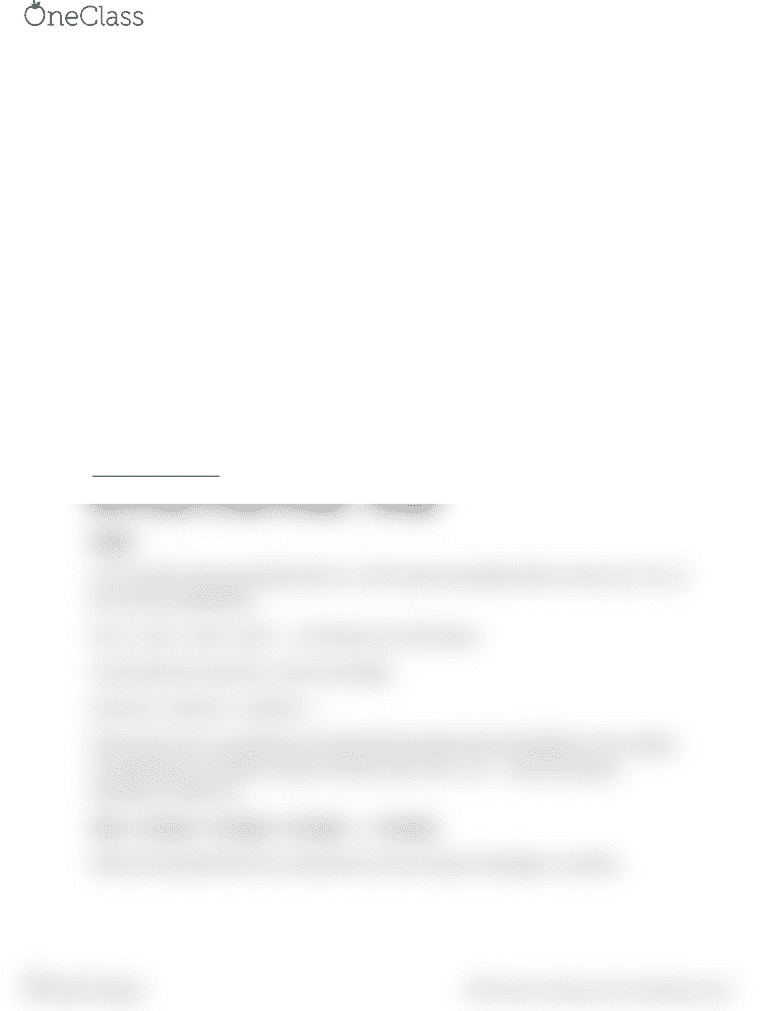# MATH 302 Lecture Notes - Lecture 4: Random Variable

20 views1 pages
School
Department
CourseExpected value of a random variable
Definition: Expectation in simplest terms is taking each value, multiplying it by its
probability and then adding all the results.
How is that useful? Usually you need to find in questions related to profit loss, or
insurance policy, about how much loss or profit you would make in the long run. So for
example, if you play a game in which you win \$10 if you roll 1 on a dice. You have to pay
\$2 to play the game. Is that fair?
(10) * Pr(Rolling a 1) - (2)*(Not rolling a 1) = (10* (1/6)) – (2* (5/6)) = 0
This is a fair game, where you will break even in the long run.
So, the idea of expectation is based on experimental probability. That is repeat an
experiment a large number of times, and see the expected gains/losses.
Expectation is represented by E(X)
Formal definition
E(X) = x1*p(x1) + x2*p(x2) + x3*p(x3) … + xk*p(xk)
Proof:
Let n1 be the value associated with x1, n2 the value associated with x2 and so on. X1, x2
etc are the probabilities.
X1n1 + x2n2 + x3n3 + x4n4 … = the total sum of all values
If we divide this result by n, then we will get:
X1(n1/n) + x2(n2/n) + x3(n3/n) ….
Notice that n1/n is actually the concept behind experimental probability. If you repeat
an experiment an infinite number of times, then n1/n = p1 … hence the above
expression reduces to
E(X) = x1*p(x1) + x2*p(x2) + x3*p(x3) … + xk*p(xk)
Notice the parallel that this concept has to the concept of average in numbers.
find more resources at oneclass.com
find more resources at oneclass.com
Unlock document

This preview shows half of the first page of the document.
Unlock all 1 pages and 3 million more documents.

Already have an account? Log in

# Get access

Grade+
\$10 USD/m
Billed \$120 USD annually
Homework Help
Class Notes
Textbook Notes
40 Verified Answers
Study Guides
1 Booster Class
Class+
\$8 USD/m
Billed \$96 USD annually
Homework Help
Class Notes
Textbook Notes
30 Verified Answers
Study Guides
1 Booster Class